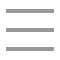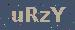个人随笔

RSA 非对称加密原理
2020-04-06 23:33:06

#### RSA 加密原理

1 找出质数 P 、Q 质数也是素数，是除了1和它本身不在有别的因数
2 计算公共模数 N = P * Q -
3 欧拉函数 φ(N) = (P-1)(Q-1) -
4 计算公钥E 1 < E < φ(N) E的取值必须是整数E 和 φ(N) 必须是互质数
5 计算私钥D E * D = 1 (mod φ(N) ) E*D 对 φ(N)取余
6 加密 C ＝ M ^E ( mod N) C：密文 M：明文
7 解密 M ＝C^ D (mod N) C：密文 M：明文

#### 第四步骤的互质数是啥？

1. 任意两个质数构成互质关系，比如13和61。

2. 一个数是质数，另一个数只要不是前者的倍数，两者就构成互质关系，比如3和10。

3. 如果两个数之中，较大的那个数是质数，则两者构成互质关系，比如97和57。

4. 1和任意一个自然数是都是互质关系，比如1和99。

5. p是大于1的整数，则p和p-1构成互质关系，比如57和56。

6. p是大于1的奇数，则p和p-2构成互质关系，比如17和15。

#### 举个例子

##### 第二步，计算p和q的乘积n。

``n = 61×53 = 3233``

n的长度就是密钥长度。3233写成二进制是110010100001，一共有12位，所以这个密钥就是12位。实际应用中，RSA密钥一般是1024位，重要场合则为2048位。

##### 第三步，计算n的欧拉函数φ(n)。

`` φ(n) = (p-1)(q-1)``

##### 第五步，计算e对于φ(n)的模反元素d。

``ed ≡ 1 (mod φ(n))``

`` ed - 1 = kφ(n)``

``ex + φ(n)y = 1``

``17x + 3120y = 1``

#### RSA算法的可靠性

p
q
n
φ(n)
e
d

（1）ed≡1 (mod φ(n))。只有知道e和φ(n)，才能算出d。

（2）φ(n)=(p-1)(q-1)。只有知道p和q，才能算出φ(n)。

（3）n=pq。只有将n因数分解，才能算出p和q。

“对极大整数做因数分解的难度决定了RSA算法的可靠性。换言之，对一极大整数做因数分解愈困难，RSA算法愈可靠。

假如有人找到一种快速因数分解的算法，那么RSA的可靠性就会极度下降。但找到这样的算法的可能性是非常小的。今天只有短的RSA密钥才可能被暴力破解。到2008年为止，世界上还没有任何可靠的攻击RSA算法的方式。　　假如有人找到一种快速因数分解的算法，那么RSA的可靠性就会极度下降。但找到这样的算法的可能性是非常小的。今天只有短的RSA密钥才可能被暴力破解。到2008年为止，世界上还没有任何可靠的攻击RSA算法的方式。

12301866845301177551304949
58384962720772853569595334
79219732245215172640050726
36575187452021997864693899
56474942774063845925192557
32630345373154826850791702
61221429134616704292143116
02221240479274737794080665
351419597459856902143413

33478071698956898786044169
84821269081770479498371376
85689124313889828837938780
02287614711652531743087737
814467999489
×
36746043666799590428244633
79962795263227915816434308
76426760322838157396665112
79233373417143396810270092
798736308917

#### 加密解密例子

##### （1）加密要用公钥 (n,e)

``m^e ≡ c (mod n)``

``65^17 ≡ 2790 (mod 3233)``

##### （2）解密要用私钥(n,d)

``c^d ≡ m (mod n)``

``2790^2753 ≡ 65 (mod 3233)``

#### 私钥解密的证明

``c^d ≡ m (mod n)``

``ｍ^e ≡ c (mod n)``

c = m^e - kn

``(m^e - kn)^d ≡ m (mod n)``

``m^ed ≡ m (mod n)``

``ed ≡ 1 (mod φ(n))``

``ed = hφ(n)+1``

``m^(hφ(n)+1) ≡ m (mod n)``

##### （1）m与n互质。

``m^φ(n) ≡ 1 (mod n)``

``(m^φ(n))^h × m ≡ m (mod n)``

##### （2）m与n不是互质关系。

``(kp)^(q-1) ≡ 1 (mod q)``

``[(kp)^(q-1)]^(h(p-1)) × kp ≡ kp (mod q)``

``(kp)^ed ≡ kp (mod q)``

``(kp)^ed = tq + kp``

``(kp)^ed = t'pq + kp``

``m^ed ≡ m (mod n)``

534Copyright : 个人随笔   备案号 : 粤ICP备18099399号